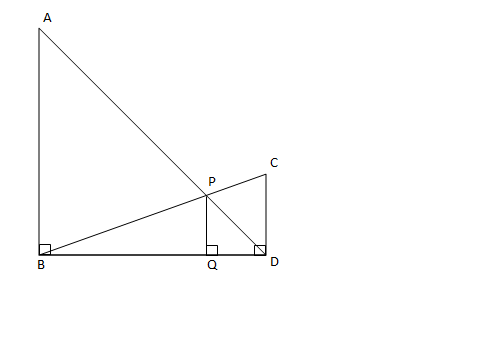Question 84

# In the diagram given below, $$\angle{ABD}$$ = $$\angle{CDB}$$ = $$\angle{PQD}$$ = 90° . If AB:CD = 3:1, the ratio of CD: PQ isSolution

Let BQ = z , QD = y , PQ = x.

From similar triangles PQD and ABD we have

(y/x) = (z+y)/3 .

Also from  similar triangles PQB and CBD we have

(z/x) = z+y .

Solving we get z = 3*y.

Now required ratio is (z+y)/z.

We get eual to 4/3 which is equal to 1:0.75.